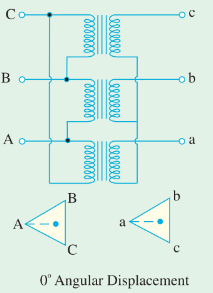WAZIPOINT Engineering Science & Technology: Transformer Delta-Delta Connection

## Friday, September 23, 2022

### Transformer Delta-Delta ConnectionFig: Delta-Delta or ∆-∆ Connection

## Delta-Delta Connection Procedure of Three-Phase Transformer in Power Transmission Network

This connection is economical for large, low-voltage transformers in which the insulation problem is not so urgent, because it increases the number of turns/phases.

The transformer connections and voltage triangles are shown in the above figure. The ratio of transformation between primary and secondary line voltage is exactly the same as that of each transformer.

Further, the secondary voltage triangle ABC occupies the same relative position as the primary voltage triangle ABC i.e., there is no angular displacement between the two.

Moreover, there is no internal phase shift between phase and line voltages on either side as was the case in Y − Y connection.

### Why is Delta-Delta Connection necessary for Three-Phase Transformer?

The major application of the Delta-Delta three-phase transformer connection in the power transmission network is as below:

Suitable for large, low-voltage transformers of the distribution system.

Delta-Delta type of connection is normally uncommon but used in some industrial facilities to reduce the impact of SLG faults on the Primary System.

Delta-Delta connection is generally used in systems where it needs to carry large currents on low voltages and especially when continuity of service is to be maintained even though one of the phases develops a fault.

### Advantages of delta-Delta three-phase transformer connection

Delta-delta connection of a three-phase transformer has the following advantages:

1. As explained above, in order that the output voltage to be sinusoidal, it is necessary that the magnetizing current of the transformer must contain a third harmonic component. In this case, the third harmonic component of the magnetizing current can flow in the ∆-connected transformer primaries without flowing in the line wires. The three phases are 120° apart which is 3 × 120 = 360° with respect to the third harmonic, hence it merely circulates in the ∆. Therefore, the flux is sinusoidal which results in sinusoidal voltages.

2. No difficulty is experienced from unbalanced loading as was the case in Y − Y connection. The three-phase voltages remain practically constant regardless of load imbalance.

3. An added advantage of this connection is that if one transformer becomes disabled, the system can continue to operate in open-delta or in V − V although with reduced available capacity. The reduced capacity is 58% and not 66.7% of the normal value.

### Disadvantages of delta-delta transformer connection

The main disadvantage of the delta-delta transformer is that there is no star-point or neutral terminal available. Therefore, the delta-delta connected transformer is used when neither primary nor secondary requires a neutral terminal and the voltages are low and moderate.